# Magnetic Field and Current Density Perturbations

Consider a tearing mode perturbation that has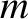periods in the poloidal direction, and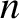periods in the toroidal direction, where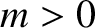,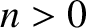, and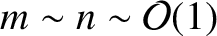. We shall assume that all perturbed scalar and vector quantities vary as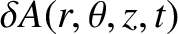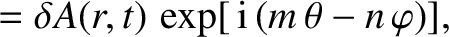(3.8)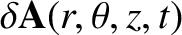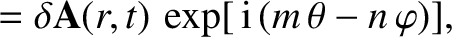(3.9)

respectively, where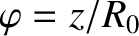is a simulated toroidal angle.

Given that tearing modes in tokamak plasmas are relatively low-amplitude (i.e.,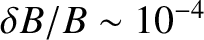) , global (i.e.,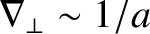) [see Equation (2.352)], relatively slowly-growing (i.e.,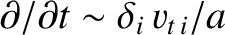) [see Equation (2.362)] instabilities, it follows from the analysis of Section 2.25 that they are governed by the linearized forms of the equations of marginally-stable ideal-MHD, (2.375)–(2.380). In particular, the linearized form of the curl of the force balance criterion, (2.377), combined with the linearized forms of Maxwell's equations, (2.349)–(2.351), give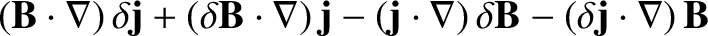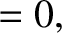(3.10)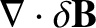(3.11)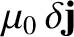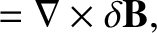(3.12)

where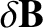and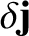are the perturbed magnetic field and current density, respectively.

Equations (3.1), (3.3), and (3.9)–(3.12) yield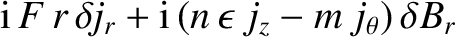(3.13)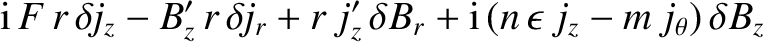(3.14)

and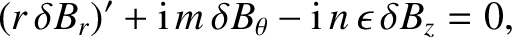(3.15)

with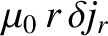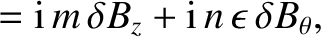(3.16)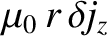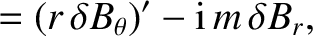(3.17)

where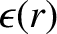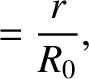(3.18)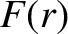(3.19)

If we write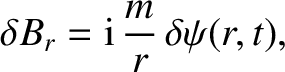(3.20)

then, after some algebra, Equations (3.13)–(3.17) reduce to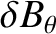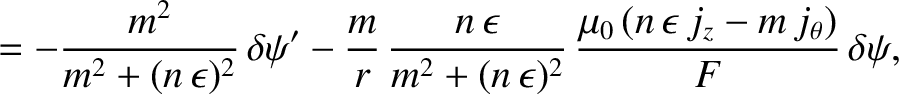(3.21)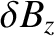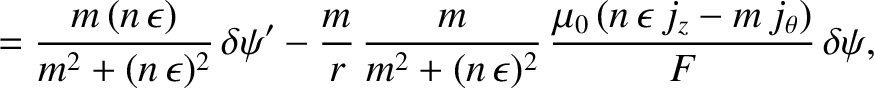(3.22)

and [6,10]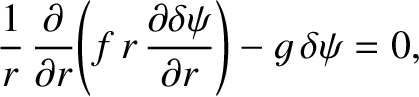(3.23)

where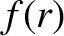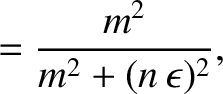(3.24)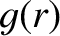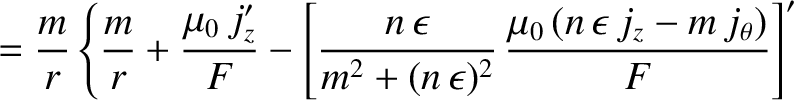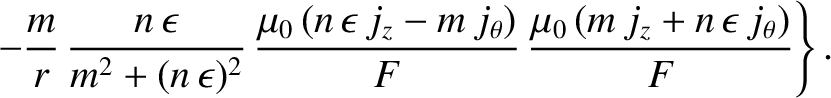(3.25)

Now, a global tearing instability in a low-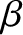, large aspect-ratio, tokamak plasma is characterized by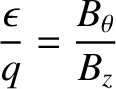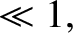(3.26)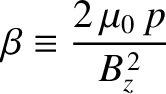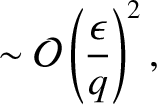(3.27)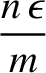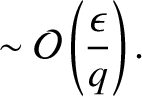(3.28)

It follows from Equations (3.4), (3.5), (3.7), and (3.19) that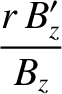(3.29)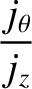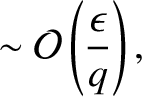(3.30)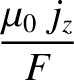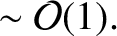(3.31)

Thus, in the low-, large aspect-ratio limit, Equations (3.20)–(3.25) simplify considerably to give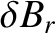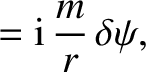(3.32)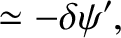(3.33)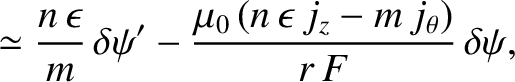(3.34)

and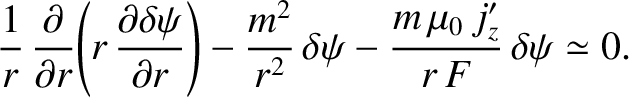(3.35)

It is also easily demonstrated that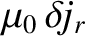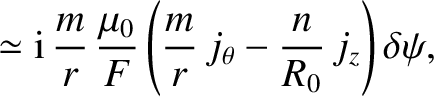(3.36)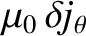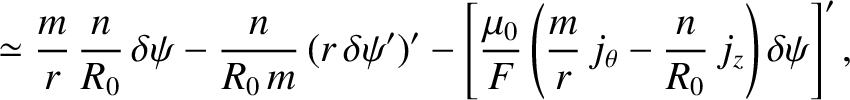(3.37)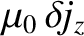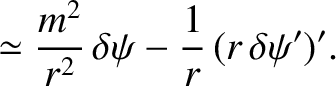(3.38)

Hence, we conclude that the magnetic field and current density perturbations associated with a tearing mode in a low-, large aspect-ratio, tokamak plasma are specified by Equations (3.32)–(3.38). From now on, we shall treat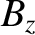as approximately independent of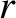, in accordance with Equation (3.29).